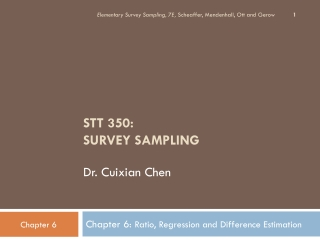DownloadDownload PresentationSTT 350: SURVEY SAMPLING Dr. Cuixian Chen

# STT 350: SURVEY SAMPLING Dr. Cuixian Chen

Télécharger la présentation## STT 350: SURVEY SAMPLING Dr. Cuixian Chen

- - - - - - - - - - - - - - - - - - - - - - - - - - - E N D - - - - - - - - - - - - - - - - - - - - - - - - - - -
##### Presentation Transcript

1. Elementary Survey Sampling, 7E, Scheaffer, Mendenhall, Ott and Gerow STT 350:SURVEY SAMPLINGDr. Cuixian Chen Chapter 6: Ratio, Regression and Difference Estimation

2. 6.2 Ratio Estimators: examples • Wholesale price paid for oranges in large shipments is based on sugar content in load. Try to estimate exact sugar content. • One method: first estimate mean sugar content per orange, my, and then to multiply by # of oranges N in load. So randomly sample n oranges from load to determine sugar content y for each. • Average of these sample measurements, yl, y2, . . . , yn, will estimate my; will estimate total sugar content for load, ty. • Unfortunately, this method is not feasible because it is too time-consuming and costly to determine N (i.e., to count total # of oranges in the load). Elementary Survey Sampling, 7E, Scheaffer, Mendenhall, Ott and Gerow

3. 6.2 Ratio Estimators: examples • We can avoid knowing Nby noting two facts: • First, sugar content of an individual orange, y, is closely related to its weight x; • Second, ratio of total sugar content ty to the total weight of the truckload tx is equal to the ratio of the mean sugar content per orange, my, to the mean weight mx. • my/mx= Nmy/Nmx= ty / tx Elementary Survey Sampling, 7E, Scheaffer, Mendenhall, Ott and Gerow

4. 6.2 Ratio Estimators: examples • Ratio estimation is used in the analysis of data from many important and practical surveys used by government, business, and academic researchers. • For instance, the CPI is actually a ratio of costs of purchasing a fixed set of items of constant quality and quantity for two points in time. • Currently, the CPI compares today’s prices with those of the 1982–1984 period. The CPI is based, in part, on data collected every month or every other month from approximately 24,000 establishments (stores, hospitals, filling stations, and so on) selected from many areas around the country. The CPI is used mainly as a measure of inflation (see Chapter 1). Elementary Survey Sampling, 7E, Scheaffer, Mendenhall, Ott and Gerow

5. 6.2 Ratio Estimators • Looking at two measurements of a population, call these x and y (we will only focus on the SRS sampling strategy) • Note that the ratio of population means is the same as the ratio of population totals!! • my/mx= Nmy/Nmx= ty / tx • Therefore, we will use • R = ratio of the means • = ratio of the totals

6. 6.3 Ratio estimator for SRS Elementary Survey Sampling, 7E, Scheaffer, Mendenhall, Ott and Gerow

7. Eg6.1, page 173 Elementary Survey Sampling, 7E, Scheaffer, Mendenhall, Ott and Gerow

8. Eg6.1, page 173 An essential rule of data analysis is to plot the data first. A scatter plot of 2002 versus 1994 data shown in Fig 6.1. if For ratio estimation to work well: Strong, positive linear trend here is important. None of data pts deviate sharply from the linear pattern. Elementary Survey Sampling, 7E, Scheaffer, Mendenhall, Ott and Gerow

9. Eg6.1, page 173 Elementary Survey Sampling, 7E, Scheaffer, Mendenhall, Ott and Gerow

10. Rewrite estimated variance of r using coefficient of variation (CV) Elementary Survey Sampling, 7E, Scheaffer, Mendenhall, Ott and Gerow

11. Ratio estimator of total Elementary Survey Sampling, 7E, Scheaffer, Mendenhall, Ott and Gerow

12. Eg6.2, page176 Elementary Survey Sampling, 7E, Scheaffer, Mendenhall, Ott and Gerow

13. Ratio estimator of Population Means Elementary Survey Sampling, 7E, Scheaffer, Mendenhall, Ott and Gerow

14. Eg6.3, page178 Elementary Survey Sampling, 7E, Scheaffer, Mendenhall, Ott and Gerow

15. Eg6.3,page178 Elementary Survey Sampling, 7E, Scheaffer, Mendenhall, Ott and Gerow

16. Ratio estimator of total and mean • For population total: • thaty = rtx • Estimated variance of thaty • N2(1-n/N) sr2 /n (where sr2 is defined previously) • For population mean: • mhaty = rmx • Estimated variance of mhaty • (1-n/N) sr2 /n (where sr2 is defined previously)

17. EX6.1, page 204 Elementary Survey Sampling, 7E, Scheaffer, Mendenhall, Ott and Gerow

18. EX6.1, page 204 • mean of x (square-foot) = 0.558333 mean of y ( volume) = 11.83333 thaty = (11.8333/.558333)*75=1589.552204 sr2 = 1.750299 Estimated variance = 250^2*(1-12/250)* 1.750299/12 = 8678.566 Bound=2*sqrt(8678.566) = 186.3176 Without fpc (don’t really need it since 1-n/N = 0.952), B = 190.957

19. EX6.1: R codes R-code: ## After download the Excel file as EX6.1.csv to the desktop dat=read.csv(file.choose()) attach(dat) x=Basal.ar; y=Volume xbar=mean(x); ybar=mean(y) r=ybar/xbar Tau_hat=75*r Sr2=var(y-r*x) N=250; n=12 B=2*sqrt(N^2*(1-n/N)*Sr2/n) ___________________________________________________ • For EX6.2 • rhat =250* 11.83333 = 2958.333 • Variance for that=250^2*(1-12/250)*26.87879/12 = 133274 • Bound = 730.1342 • Without fpc, bound = 748.3146

20. EX6.2, page 204 Elementary Survey Sampling, 7E, Scheaffer, Mendenhall, Ott and Gerow

21. EX6.6, page 205 Elementary Survey Sampling, 7E, Scheaffer, Mendenhall, Ott and Gerow

22. More examples • Do problem 6.6 mean(x)=16.47273,mean(y) = 16.84545, so rhat = 16.84545/16.47273=1.022627; sr2=0.2049424

23. 6.4 Sample size estimation • n = Ns2/(ND + s2) where we can estimate s2 as Si(yi-rxi)2/(n’-1) with n’ a small preliminary sample With D=B2mx2/4 for estimating R D = B2/4 for estimating my D = B2/(4N2) for estimating ty

24. 6.4 Sample size estimation for SRS

25. Eg6.4, page 180 n’ Elementary Survey Sampling, 7E, Scheaffer, Mendenhall, Ott and Gerow

26. Eg6.5, page 182 Elementary Survey Sampling, 7E, Scheaffer, Mendenhall, Ott and Gerow

27. Eg6.6, page 184 Elementary Survey Sampling, 7E, Scheaffer, Mendenhall, Ott and Gerow

28. EX6.13, page 207 Elementary Survey Sampling, 7E, Scheaffer, Mendenhall, Ott and Gerow